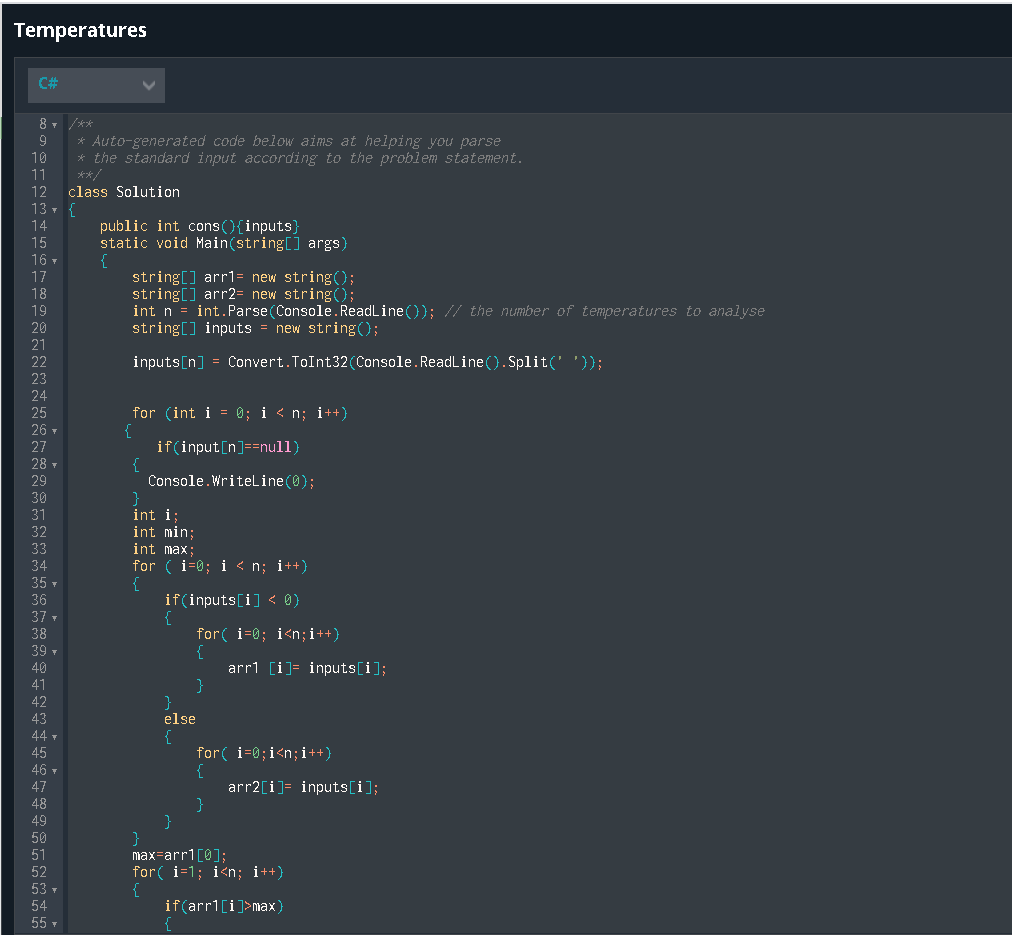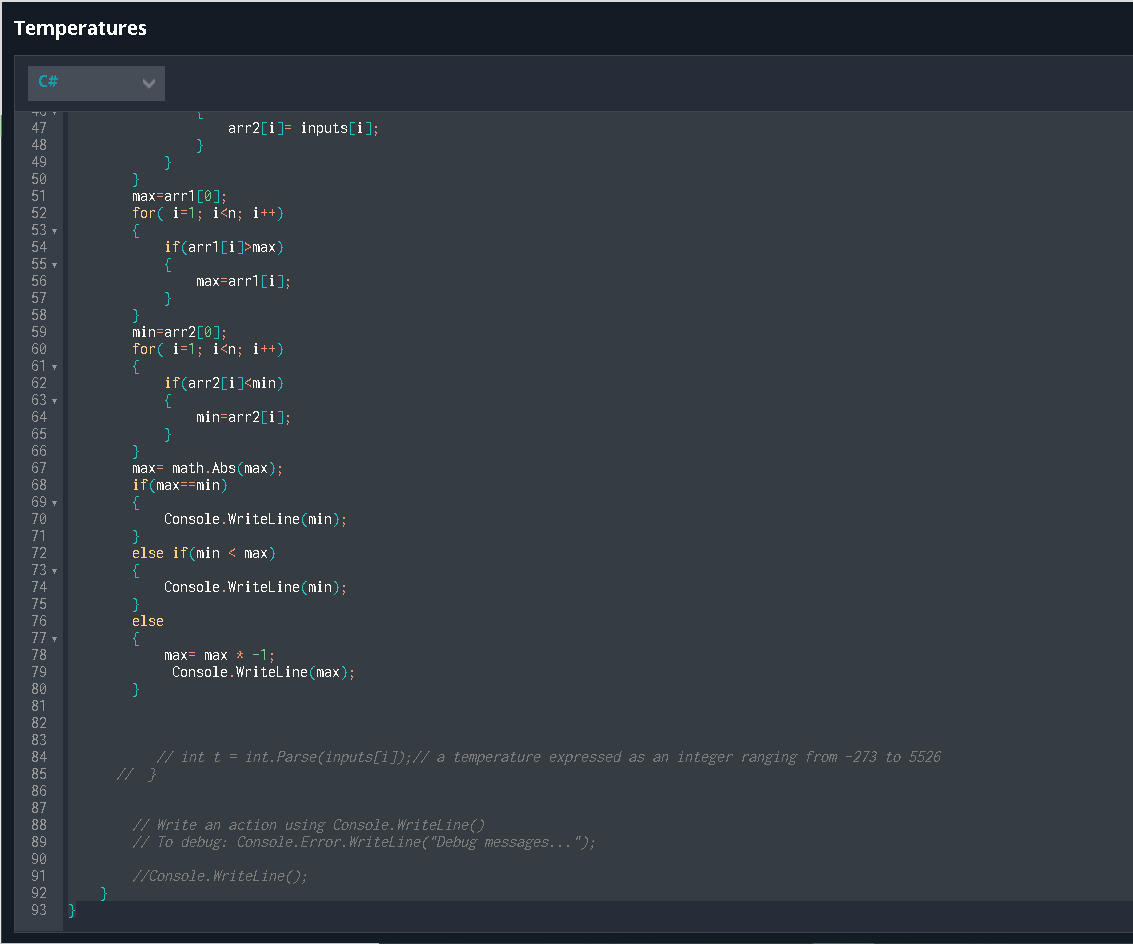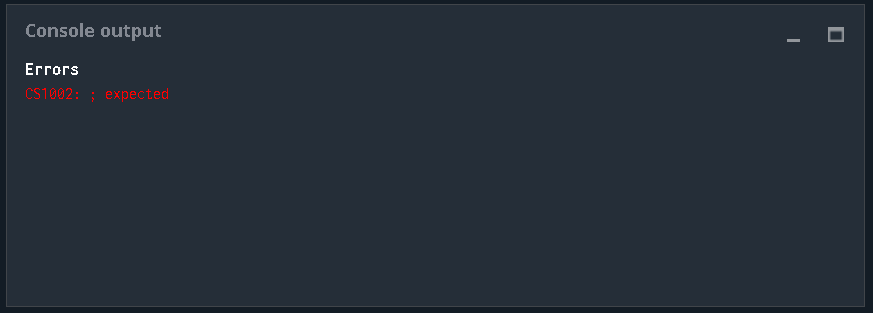# Temperatures puzzle discussion

#781
``````import sys
import math

# Auto-generated code below aims at helping you parse
# the standard input according to the problem statement.

n = int(input())# the number of temperatures to analyse
x = 0
tpos = []
tneg = []
mmm = False
nnn = False

for i in input().split():
# t: a temperature expressed as an integer ranging from -273 to 5526
t = int(i)
if x < t :
tpos = [t]
elif x > t :
tneg = [t]

if len(tpos) > 0 :
m = min(tpos)
mmm = True
elif len(tpos) == 0 :
mmm = False

if len(tneg) > 0 :
n = max(tneg)
nnn = True
elif len(tneg) == 0 :
nnn = False

if nnn == True and mmm == True :
mm = m*m
nn = n*n
if nn > mm :
result = m
elif mm > nn :
result = n
elif mm == nn :
result = m
if nnn == True and mmm == False :
result = max(tneg)

if nnn == False and mmm == True :
result = min(tmax)

if nnn == False and mmm == False :
result = 0
# Write an action using print
print("Debug messages...", file=sys.stderr)

print(result)
``````

#782

Insert after the first “for”

print(tpos,file=sys.stderr)
print(tneg,file=sys.stderr)

After i think that you’ll easily find where is the problem …#784

Am i even on the right path?

class Solution
{
public int cons(){inputs}
static void Main(string[] args)
{
string[] arr1= new string();
string[] arr2= new string();
int n = int.Parse(Console.ReadLine()); // the number of temperatures to analyse
string[] inputs = new string();

``````    inputs[n] = Convert.ToInt32(Console.ReadLine().Split(' '));
if(input[n]==null)
{
Console.WriteLine(0);
}
int i;
int min;
int max;
for ( i=0; i < n; i++)
{
if(inputs[i] < 0)
{
for( i=0; i<n;i++)
{
arr1 [i]= inputs[i];
}
}
else
{
for( i=0;i<n;i++)
{
arr2[i]= inputs[i];
}
}
}
max=arr1;
for( i=1; i<n; i++)
{
if(arr1[i]>max)
{
max=arr1[i];
}
}
min=arr2;
for( i=1; i<n; i++)
{
if(arr2[i]<min)
{
min=arr2[i];
}
}
max= math.Abs(max);
if(max==min)
{
Console.WriteLine(min);
}
else if(min < max)
{
Console.WriteLine(min);
}
else
{
max= max * -1;
Console.WriteLine(max);
}
``````

}
}

#785

The problem appears to be that you’re using tpos = [t] and tneg = [t], on lines 18 and 20 respectively, which isn’t actually appending the lists.

#786

i’m lost#787

line 14 doesn’t make sense

#788

you wrote this :
t = int(i)
if x < t :
tpos = [t]
elif x > t :
tneg = [t]

instade of it you can write this :

``````t = int(i)
if x < t :
tpos.append(t)
elif x > t :
tneg.append(t)``````

#789

An error occurred (#73): “Only 1 executor running at the same time for a test session”. What can i do?

#790

#791

I don’t know why my variable is not always responding to my if test.
I’m trying to solve in java script and have written this code after the code provided initialy:

``````let posT=5526, negT= -273, T=null;

for (let i = 0; i < n; i++) {
if (inputs[i]>=0 && inputs[i] < posT) {posT=inputs[i]}
if (inputs[i]<0 && inputs[i] > negT) {negT=inputs[i]}
console.error(posT);
}

if (posT <= -negT) {T=posT}
else if (posT > -negT) {T=negT}

console.error(".");

console.log(T);

console.error(".");
for (let i = 0; i < n; i++) {console.error(inputs[i])}
console.error(".");
console.error(posT);
console.error(negT);
``````

and this is my console output:

``````### Console output

### Standard Output Stream:

42

42

12

12

12

12

.

-5

.

42

5

12

21

-5

24

.

12

-5

### Failure

Found:

-5

Expected:

5
``````

I was expecting my posT variable to be 5 at i=1 on the for loop, but it’s not working,but it does works for some other values. That also happens with negT on other tests

#792

already found it, the input given were strings instead of numbers, so the comparison was bugged. Solved it with a few changes to get 100% score

#793

So thats my Code on C# i only got a score of 81% and i dont know why can anyone help me?

``````using System;
using System.Linq;
using System.IO;
using System.Text;
using System.Collections;
using System.Collections.Generic;

/**
* Auto-generated code below aims at helping you parse
* the standard input according to the problem statement.
**/
class Solution
{
static void Main(string[] args)
{

int n = int.Parse(Console.ReadLine()); // the number of temperatures to analyse

int temp = 0; // Ausgegebene Temperatur
int maxP = 5526; // Höchsttemperatur Plus
int maxM = -273; // Höchsttemperatur minus
int Null = 0;

for (int i = 0; i < n; i++)
{
int t = int.Parse(inputs[i]);// a temperature expressed as an integer ranging from -273 to 5526

Console.Error.WriteLine(inputs[i]);

if ( (Null < t) & (t < maxP) & (maxM * -1 >= t))
{
maxP = t;
temp = maxP;
}
else if ( (Null > t) & (t > maxM) & (maxP * -1 <= t))
{
maxM = t;
temp = maxM;
}

}

// Write an action using Console.WriteLine()
// To debug: Console.Error.WriteLine("Debug messages...");
if ( temp != 0)
{
if (maxP * -1 == temp)
{
Console.WriteLine(maxM * -1);
}
else
{
Console.WriteLine(temp);
}

}
else
{

Console.WriteLine(0);
}
}
}``````

#794

you fail these two validators:Does your code manage when there is only one temperature in input (for example, -273 or 2256) ?

#795

Oh now I get it thanks for the help

#796

I’ll take it back I don’t understand it, can you explain it to me in more detail?

#797

if I give only 1 temperature to your code in input, what does it return?

#798

It outputs the entered temperature

#799

not for all temperatures it seems#800

Oh now I see the mistake …
Well now I know why it doesn’t work. but I have an idea, let’s see if anything changes.

#801

Hey, i am very grateful for your help.
Without you, I really wouldn’t have got the 100% score.
Thank you